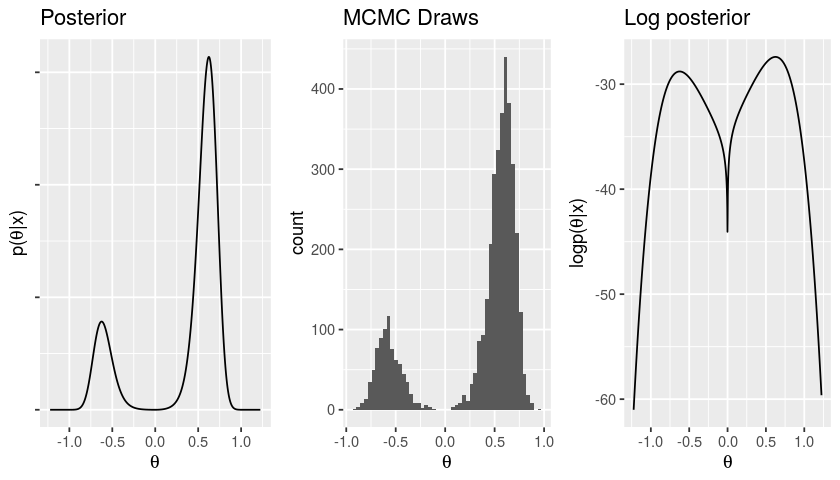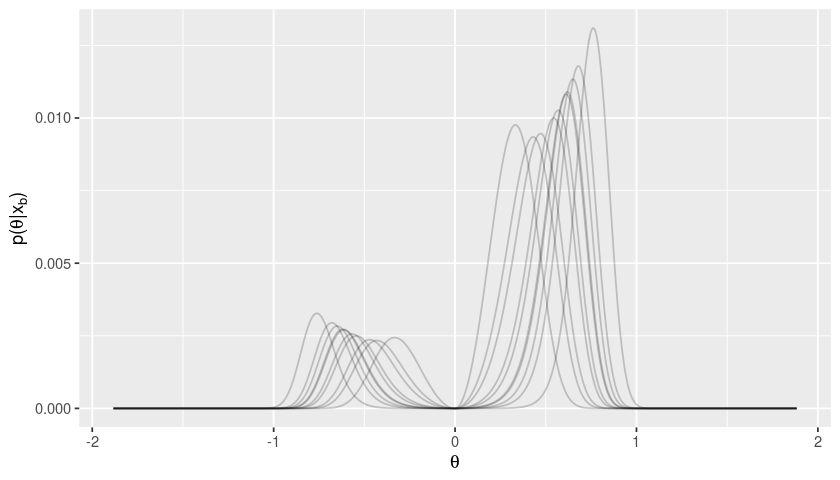The following post is generated from this Jupyter notebook. Forgive the formatting — I’m still working out how best to post notebooks.

bayesian_bootstrap_v1

# The Bayesian Bootstrap Is Not a Free Lunch¶

Some recent work has suggested that we can perform computationally difficult, multi-modal Bayesian posterior calculations with optimization and bootstrap sampling (see Fong (2019), for example).

The basic idea can take many forms, but it's always based on something similar to considering draws $\theta_b = \mathrm{argmax}_\theta p(\theta | x_b)$, where $x_b$ are bootstrap samples of the original data $x$. The draws $x_b$ may not be standard bootstrap samples (e.g. they may use Dirichlet rather than multinomial weights), and the objective function may not be precisely $p(\theta | x)$ (e.g. it may be $p(x | \theta)$), but these differences will not be material for the purposes of this post. I will refer to this basic idea as the "Bayesian bootstrap" (BB) with the awareness that the BB encompasses many variants.

There are many good reasons to be interested in the distribution of $x_b$. For example, you might want to know the frequentist properties of the posterior, you might be intersted in the performance of the posterior over many similar datasets, you might actually want the minimizer of an unknown loss function on which you have a Dirichlet process prior, you might want proposals for importance sampling, you might just want a heuristic summary statistic, etc. However, it is misguided in general to hope that draws of $\theta_b$ are a good substitue for draws $\theta \sim p(\theta | x)$ from the true posterior when $p(\theta | x)$ is known.

The shortfall is arguably clearest when the posterior is multimodal, a case when the BB might superfically show the most promise. Sampling from multimodal posteriors is hard in general and the BB may be a better solution than none at all. However, the BB is most useful as an approximate solution when its shortcomings are clearest. In this post I hope to illustrate how the BB can go wrong as a posterior approximation on a very simple toy example.

### Model¶

Let us consider a toy model which is simple and contrived. Its simplicity is such that we can clearly see what the right Bayesian answer should be, and how the BB fails to replicate it. I hope to argue in future, less simple examples that its contrivance is not so severe, and that the failure of the BB in this simple model illustrate its potential failures in more complex cases.

Suppose we observe a vector of scalar, real-valued data $x=(x_1,...,x_N)$, drawn from the following model:

\begin{align} p(\tau) &= \mathrm{Gamma}(\tau | 1, 1)\\ p(x | \tau) &= \prod_{n=1}^N \mathcal{N}(x_n | \tau, 1). \end{align}

The unkown mean parameter $\tau$ is positive, and all other aspects of the data generating process are known. As stated, this is a simple problem for which MCMC works perfectly well.

Now, let us suppose that we are actually interested in a quantity $\theta \in \mathbb{R}$ which is the signed square root of $\tau$, with the probability of being positive and negative specified by the prior.

\begin{align} z &\in \{-1, 1\}\\ p(z=1) &= 0.8\\ \theta &= z \sqrt{\tau}. \end{align}

The variable $z$ denotes the sign of $\theta$, and $\theta^2 = \tau$. The posterior probability that $\theta$ is positive is $p(\theta > 0 | x) = 0.8$ because the data $x$ is independent of $z$, the sign of $\theta$. Effectively, the sign of $\theta$ is identified only by the prior, and the posterior $p(\theta \vert x)$ has both a positive mode and a negative mode, which it occupies with respective probabilities $0.8$ and $0.2$. We can sample from the posterior of $p(\theta | x)$ by sampling from $p(\tau | x)$, drawing $z$ from its prior, and setting $\theta = z \tau$.

Can the BB reproduce a reasonable approximation of the posterior $p(\theta | x)$ in this trivial case? Below, I will show that it cannot. The reasons are twofold and general. Recall that the probability probability of a lying near a particular mode is determined by the posterior mass under the mode. For example, in the present case, $p(\theta > 0 | x) = \int_{0}^{\infty} p(\theta | x) d\theta = 0.8$. However, in general,

• The frequency with which an optimum finds a mode is not, in general, determined by the relative mass of a mode and
• The variability of an optimum induced by the bootstrap is not, in general, determined by the relative mass of a mode.

Let us run some simulations in R .

In :
library(tidyverse)
library(rstan)
library(gridExtra)
library(numDeriv)
library(repr)
library(latex2exp)

options(repr.plot.width=7, repr.plot.height=4)

options(mc.cores=4)
rstan_options(auto_write=TRUE)

── Attaching packages ─────────────────────────────────────── tidyverse 1.2.1 ──
✔ ggplot2 3.2.0     ✔ purrr   0.3.2
✔ tibble  2.1.3     ✔ dplyr   0.8.3
✔ tidyr   0.8.3     ✔ stringr 1.4.0
✔ readr   1.3.1     ✔ forcats 0.4.0
── Conflicts ────────────────────────────────────────── tidyverse_conflicts() ──
rstan (Version 2.19.2, GitRev: 2e1f913d3ca3)
For execution on a local, multicore CPU with excess RAM we recommend calling
options(mc.cores = parallel::detectCores()).
To avoid recompilation of unchanged Stan programs, we recommend calling
rstan_options(auto_write = TRUE)

Attaching package: ‘rstan’

The following object is masked from ‘package:tidyr’:

extract

Attaching package: ‘gridExtra’

The following object is masked from ‘package:dplyr’:

combine



Here's the model in Stan. We'll draw $p(\tau | x)$, which will mix well, and use those draws together with the prior $p(z)$ to get draws from $p(\theta, z | x)$.

In :
model_code <- "
data {
int<lower=0> N;
real x[N];
real<lower=0> x_sd;
real<lower=0, upper=1> prob;
real<lower=0> tau_prior_shape;
real<lower=0> tau_prior_rate;
}
parameters {
real<lower=0> tau;
}
model {
tau ~ gamma(tau_prior_shape, tau_prior_rate);
x ~ normal(tau, x_sd);
}
generated quantities {
real theta;
int z;
z = 2 * bernoulli_rng(prob) - 1;
theta = z * sqrt(tau);
}"

# cat(scan("multimodal_v1.stan", what="character", sep="\n"), sep="\n")


Let's draw some data and get a stanfit object.

In :
DrawAndFit <- function(N, z_prob, tau_prior_shape=1, tau_prior_rate=1, x_sd=1) {
return(list(fit=fit, bb_data=bb_data))
}

In :
N <- 50

tau_prior_shape <- 1
tau_prior_rate <- 1
x_sd <- 1
z_prob <- 0.8

tau_true <- rgamma(1, shape=tau_prior_shape, rate=tau_prior_rate)
x <- rnorm(N, mean=tau_true, sd=x_sd)

# Also save tau_true even though Stan doesn't use it.
bb_data <- list(x=x, N=N, prob=z_prob, x_sd=x_sd,
tau_prior_shape=tau_prior_shape,
tau_prior_rate=tau_prior_rate,
tau_true=tau_true)

# The Stan model will exhibit some divergent transitions near 0, but that's
# not a real problem here.
initial_fit <- stan(model_code=model_code, data=bb_data)


Let's take a look at the posterior of $\theta$.

In :
EvalLogPost <- function(theta, fit, z_prob) {
# Use the stanfit object to evaluate the log posterior as a
# function of theta.

upars <- unconstrain_pars(fit, list(tau=theta ^ 2))

# The Stan posterior doesn't contain the prior for z so add it in.
lp <- log_prob(fit, upars) + ifelse(theta > 0, log(z_prob), log(1 - z_prob))
return(lp)
}

GetThetaGridLp <- function(fit, z_prob, theta_max=NA) {
# Evaluate the log posterior at a grid of theta values for plotting.

draws <- extract(fit)
if (!is.finite(theta_max)) {
theta_max <- 1.3 * max(abs(draws$theta)) } theta_grid <- seq(-1 * theta_max, theta_max, length.out=1000) lp <- sapply(theta_grid, EvalLogPost, fit, z_prob) return(data.frame(theta=theta_grid, lp=lp)) }  In : draws <- extract(initial_fit) cat("P(\\theta > 0) \\approx ", mean(draws$theta > 0), "\n")

theta_lp <- GetThetaGridLp(initial_fit, z_prob)
grid.arrange(
ggplot(theta_lp) +
geom_line(aes(x=theta, y=exp(lp))) +
ggtitle("Posterior") + xlab(TeX("$\\theta$")) + ylab(TeX("$p(\\theta | x)$")) +
scale_y_continuous(labels=NULL)
,
ggplot() +
geom_histogram(aes(x=draws$theta), bins=50) + ggtitle("MCMC Draws") + xlab(TeX("$\\theta$")) , ggplot(theta_lp) + geom_line(aes(x=theta, y=lp)) + ggtitle("Log posterior") + xlab(TeX("$\\theta$")) + ylab(TeX("$\\log p(\\theta | x)$")) , ncol=3 )  P(\theta > 0) \approx 0.79525Now, let's see whether the Bayesian bootstrap will reproduce$p(\theta > 0 | x) = 0.8$. First of all, note that reasonable optimizers (e.g. well-conditined gradient ascent) will find the positive mode if the initial value is positive, and the negative mode if the initial value is negative. So, irrespective of the noise induced by the bootstrap, the BB estimate of$P(\theta > 0)$will be precisely the probability that the initial value for optimization is positive. With symmetric random restarts, this probability will be$0.5$, not$0.8$. There is no need to run simulations to confirm this result. A resonable question is whether drawing bootstrap samples of$x$and finding a global optimum can overcome this obvious shortcoming. Let's take a look. In : DrawBootstrapSample <- function(x) { return(x[sample.int(length(x), replace=TRUE)]) } GetBootstrapFit <- function(bb_data) { x_boot <- DrawBootstrapSample(bb_data$x)
bb_data_boot <- bb_data
bb_data_boot$x <- x_boot # A stanfit object will allow us to evaluate the log probability at # the bootstrapped data. # # We only need the fit object, not the draws, so set samples to be very low and # suppress output and warnings. options(warn=-1) invisible(capture.output( fit_boot <- stan(model_code=model_code, data=bb_data_boot, iter=1, chains=1))) options(warn=0) return(list(x_boot=x_boot, fit_boot=fit_boot)) }  In : theta_max <- 2 * max(abs(draws$theta))
mean_abs_theta <- mean(abs(draws$theta)) # Draw bootstrap samples and save the log posteriors at the bootstrapped data. boot_lp_df <- data.frame() for (b in 1:10) { print(b) boot_fit <- GetBootstrapFit(bb_data) theta_lp <- GetThetaGridLp(boot_fit$fit, z_prob, theta_max)
boot_lp_df <- bind_rows(boot_lp_df, theta_lp %>% mutate(b=as.character(b)))
}

 1
 2
 3
 4
 5
 6
 7
 8
 9
 10


As we can see, the bootstrap variability is never such that the lower mode is larger than the higher mode. Consequently, if our optimization method finds the global optimum rather than a local optimum, it will estimate $p(\theta > 0 | x_b) = 1$.

In :
boot_lp_df <-
boot_lp_df %>%
group_by(b) %>%
mutate(lp_norm=lp - log(sum(exp(lp)))) # Were this to be a density you would need the delta x

ggplot(boot_lp_df) +
geom_line(aes(x=theta, y=exp(lp_norm), group=b), alpha=0.2) +
xlim(-1 * theta_max, theta_max) +
xlab(TeX("$\\theta$")) + ylab(TeX("$p(\\theta | x_b)$"))Let's check this formally by actually optimizing a number of bootstrap samples.

In :
GetMAP <- function(fit, init_val=NA, width=NA, pos=TRUE) {
# init_val and width are in the log space.
x_sign <- (sign(pos) * 2 - 1)
Objective <- function(log_x) {
x <- x_sign * exp(log_x)
# The log probability doesn't matter because we're only finding the optimum in
# one of the two modes.
return(-1 * EvalLogPost(x, fit, 0.5))
}
if (!is.finite(init_val)) {
init_val  <- log(mean(sqrt(extract(fit, "tau")$tau))) } if (!is.finite(width)) { width <- 4 * sd(log(sqrt(extract(fit, "tau")$tau)))
}
opt <- optim(init_val, Objective, method="Brent",
upper=init_val + width, lower=init_val - width)
opt$par_theta <- x_sign * exp(opt$par)
return(opt)
}

GetBootstrapResult <- function(fit_boot, z_prob, init_val=NA, width=NA) {
boot_opt <- GetMAP(fit_boot, pos=TRUE, init_val=init_val, width=width)
lp_neg <- EvalLogPost(-1 * boot_opt$par_theta, fit_boot, z_prob) lp_pos <- EvalLogPost(boot_opt$par_theta, fit_boot, z_prob)
lp_hess_neg <- hessian(
function(x) EvalLogPost(x, fit_boot, z_prob),
-1 * boot_opt$par_theta)[1, 1] lp_hess_pos <- hessian( function(x) EvalLogPost(x, fit_boot, z_prob), boot_opt$par_theta)[1, 1]

return(data.frame(
theta_opt=ifelse(lp_pos > lp_neg, 1, -1) * boot_opt$par_theta, lp_pos=lp_pos, lp_neg=lp_neg, lp_hess_neg=lp_hess_neg, lp_hess_pos=lp_hess_pos )) }  In : init_val <- log(mean(sqrt(draws$tau)))
width <- 4 * sd(log(sqrt(draws$tau))) B <- 50 boot_df <- data.frame() boot_time <- Sys.time() # Draw bootstrap samples and find the MAP. for (b in 1:B) { fit_boot <- GetBootstrapFit(bb_data)$fit_boot
boot_df <- bind_rows(
boot_df,
GetBootstrapResult(fit_boot, z_prob, init_val=init_val, width=width))
}
boot_time <- Sys.time() - boot_time

In :
cat("BB estimate of the probability that theta's sign is positive: ", mean(boot_df$theta_opt > 0), "\n")  BB estimate of the probability that theta's sign is positive: 1  ### Summary¶ In summary, there are two flavors of the BB: one which finds a local optimum, and one which finds a global optimum. The former estimates$p(\theta > 0 | x) \approx 0.5$, and the latter estimates$p(\theta > 0 | x) \approx 1.0$. Neither is a good estimate of the true$p(\theta > 0 | x) = 0.8\$.

What has gone wrong for the BB? The value chosen by the BB depends on two things:

• The domain of attraction and the distribution of the starting point
• The variability of the height of the optima under data resampling.

Neither of these quantites necessarily have anything to do with the real posterior probability, which is the mass under the mode.